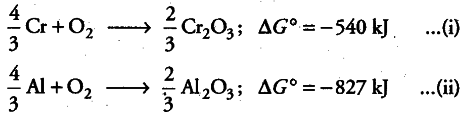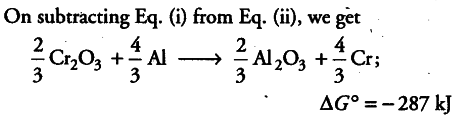# Is the reduction of \$Cr_{2}O_{3}\$ possible with Al?

The value of \$\Delta \$fG° for the formation of \$Cr_{2}O_{3}\$ is - 540 kJ \${{mol}^{-1}}\$ and that of \$Al_{2}O_{3}\$ is - 827 kJ \${{mol}^{-1}}\$. Is the reduction of \$Cr_{2}O_{3}\$ possible with Al?

The two thermochemical equations may be written as:As \$\Delta \$fG° comes out to be negative, the reaction is feasible, i.e. Al can be used for the reduction of Cr_{2}O_{3}.